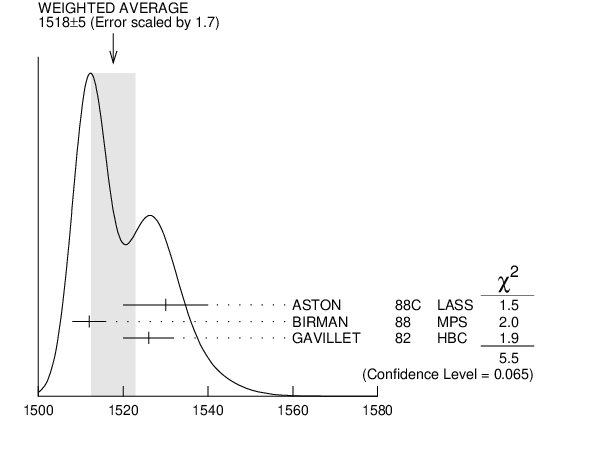# ${{\boldsymbol f}_{{1}}{(1510)}}$ MASS INSPIRE search

VALUE (MeV) EVTS DOCUMENT ID TECN  COMMENT
$\bf{ 1518 \pm5}$ OUR AVERAGE  Error includes scale factor of 1.7.
$1530$ $\pm10$
 1988 C
LASS 11 ${{\mathit K}^{-}}$ ${{\mathit p}}$ $\rightarrow$ ${{\mathit K}_S^0}$ ${{\mathit K}^{\pm}}{{\mathit \pi}^{\mp}}{{\mathit \Lambda}}$
$1512$ $\pm4$ 600 1
 1988
MPS 8 ${{\mathit \pi}^{-}}$ ${{\mathit p}}$ $\rightarrow$ ${{\mathit K}^{+}}{{\overline{\mathit K}}^{0}}{{\mathit \pi}^{-}}{{\mathit n}}$
$1526$ $\pm6$ 271
 1982
HBC 4.2 ${{\mathit K}^{-}}$ ${{\mathit p}}$ $\rightarrow$ ${{\mathit \Lambda}}{{\mathit K}}{{\mathit K}}{{\mathit \pi}}$
• • • We do not use the following data for averages, fits, limits, etc. • • •
$\sim$$1525$ 2
 1993 B
${{\mathit \gamma}}$ ${{\mathit \gamma}^{*}}$ $\rightarrow$ ${{\mathit \pi}^{+}}{{\mathit \pi}^{-}}{{\mathit \pi}^{0}}{{\mathit \pi}^{0}}$
1  From partial wave analysis of ${{\mathit K}^{+}}{{\overline{\mathit K}}^{0}}{{\mathit \pi}^{-}}$ state.
2  Not seen by AIHARA 1988C in the ${{\mathit K}_S^0}$ ${{\mathit K}^{\pm}}{{\mathit \pi}^{\mp}}$ final state.${{\mathit f}_{{1}}{(1510)}}$ mass (MeV)
References:
 BAUER 1993B
PR D48 3976 Evidence for Spin-One Resonance Production in the Reaction ${{\mathit \gamma}}$ ${{\mathit \gamma}^{*}}$ $\rightarrow$ ${{\mathit \pi}^{+}}{{\mathit \pi}^{-}}{{\mathit \pi}^{0}}{{\mathit \pi}^{0}}$
 ASTON 1988C
PL B201 573 Evidence for Two Strangeonium Resonances with $\mathit J{}^{PC} = 1++$ and $1+{}^{-}{}^{}$ in ${{\mathit K}^{-}}{{\mathit p}}$ Interactions at 11 ${\mathrm {GeV/}}\mathit c$
 BIRMAN 1988
PRL 61 1557 Partial Wave Analysis of the ${{\mathit K}^{+}}{{\overline{\mathit K}}^{0}}{{\mathit \pi}^{-}}$ System
 GAVILLET 1982
ZPHY C16 119 Evidence for a New ${{\overline{\mathit K}}}$ State at a Mass of 1530 MeV with $\mathit I(\mathit J{}^{PC}) = 0(1{}^{++})$ Observed in ${{\mathit K}^{-}}{{\mathit p}}$ Interactions at 4.2 ${\mathrm {GeV/}}\mathit c$